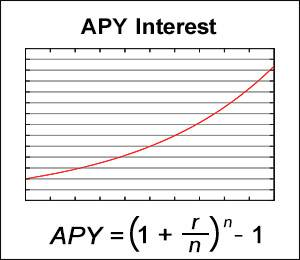# APY Interest Calculator

APY(Annual Percentage Yield) is the rate of interest earned from an investment in a year. This calculator calculates the amount of interest earned from a deposit with a given APY for a given term and can also take into account continuous monthly deposits.

For compounding times greater than 12 times/year, the calculator calculates the balance based on an equal amount of deposit added at every compounding time.

Values are rounded to the cent.

This calculator is identical to Compound Interest Calculator except for the fact that this calculator takes into account monthly deposits in addition to compounding interest.CC BY-SA 3.0 - Original Graph by Autopilot.

### Input

 Initial Deposit: \$ Annual Interest: % Years: years Compounded Times: times/year Monthly Deposit: \$